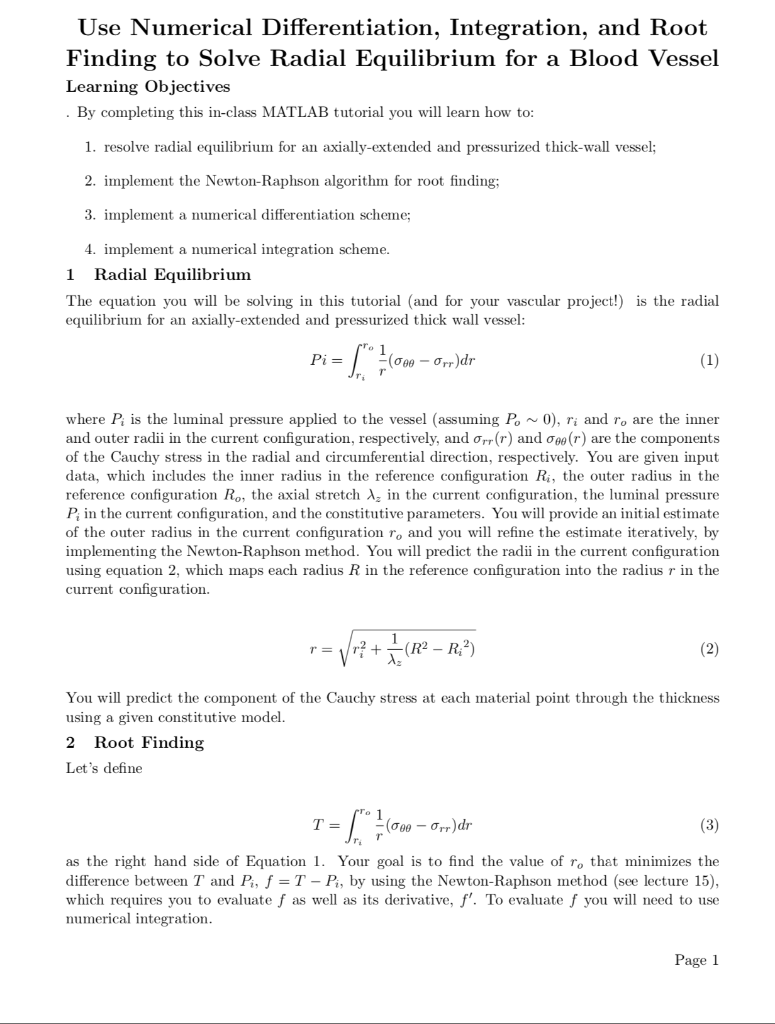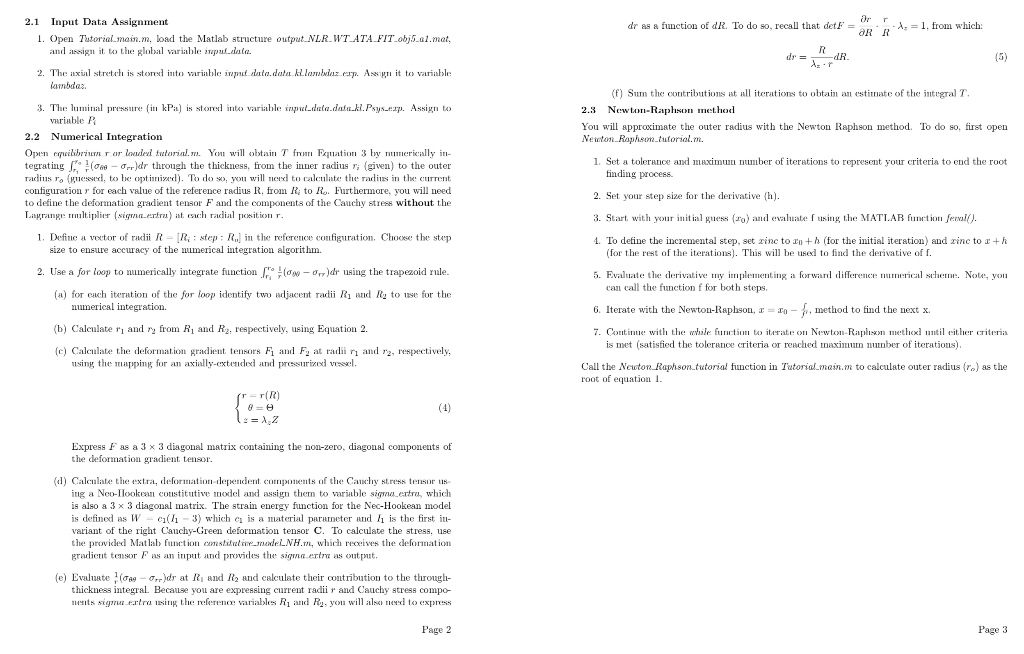Home / Expert Answers / Computer Science / below-is-my-code-error-that-i-see-while-running-the-tutotial-main-m-file-error-using-diff-dif-pa617

# (Solved): Below is my code Error that i see while running the Tutotial_main.m file Error using diff Dif ...Below is my code

Error that i see while running the Tutotial_main.m file

Error using diff
Difference order N must be a positive integer scalar.

Error in Newton_Raphson_tutorial (line 35)
f_prime0 = diff(f,x0,xinc); % compute the
derivative of f, between x0 and xinc

Error in Tutorial_m (line 51)
x = Newton_Raphson_tutorial(H,x0); % call the Newton
Raphson function (Newton_Raphson_tutorial.m)

for Tutorial_main.m

%=========================================================================

% Lecture 16: In Class Tutorial

%

% This function calculates the radial equilibrium function for an axially

% stretched and pressurized thick wall vessel and is part of the set of

% equations you will implement for your vasculature project

%

% Input data:

% luminal pressure (Pi), axial stretch (lambdaz_v)

% material parameters, radii in ktf (Ri, Ro)

%

% Output data:

% approximation of the outer radius, ro

%

% The inverse solution of the radial equilibrium involves finding

% the root of the equation:

% Pi - int_{ri}^{ro} (tqq-trr)/r dr = 0

%=========================================================================

close all, clear all, clc

%% Load and Define INPUT DATA

global input_data

% Conversion factor for Pressure: mmHg to kPa

% mmHg2kPa = 101.325/760;

% Pressure & Axial Stretch @ loaded configurations of interest

lambdaz = input_data.data_kl.lambdaz_exp; %stored in input data

Pi = input_data.data_kl.Psys_exp; %in-vivo pressure

%% Solve the equilibrium equation

% Equilibrium equation for a pressurized and axially stretched vessel

% ro will be estimated as the roots of the equilibrium equation

H=cell(1,1);

% Parameter to be estimated in loaded configuration is the outer radius (ro)

% x0 is the initial guess for ro based on the input experimental data

x0 = [input_data.data_ktf.or_exp];

input_data.data_kl.lambdaz = lambdaz; % axial stretch

input_data.data_kl.Pi = Pi; % liminal pressure, in kPa

% Newton Raphson Scheme, Inputs: function handles and evaluation points

x = Newton_Raphson_tutorial(H,x0); % call the Newton Raphson function (Newton_Raphson_tutorial.m)

or_est = x(1,1); % calculated outer radius

CODE for Newton_Raphson_tutorial.m

Doubts

According to the instructions, I have tried to define the variables.

I am not sure what should be the tolerance(tol ), step size(h).

How to define f_prime0.

2.3 point 3 to evaluate f using feval() I don't know where to write that and how to write that.

function [x] = Newton_Raphson_tutorial(H,x0)

%=========================================================================

% Newton Raphson Scheme, X_n = X_(n-1) - f/f'

%

% H is the handle to the equilibrium equation

% x0 is the initial guess for the outer radius

%

% x is the approximate solution of the Newton Raphson method (in this case,

% ro)

%=========================================================================

%% Iteration Constraints

tol = 2; % tolerance criteria to stop scheme

maxiter = 4; % maximum number of iterations allowed before stopping

%% Newton Raphson Iteration

if length(H) ~= length(x0) % check if the number of equations match the number of unknowns

disp('1Error: number of unknowns does not coincide with number of equations')

return

else

% first perform Newton Raphson for the initial guess

% evaluate the function, f, with the initial guess, x0

iter = 0; % initial iteration

h = 0.01; % step size to perform the derivative (forward difference)

xinc = x0; % initial guess of the root

xinc = xinc + h; % current root + derivative step

f_prime0 = diff(f,x0,xinc); % compute the derivative of f, between x0 and xinc

u0 = f/f_prime0; % divide f by its derivative, f/f'

x = x0-u0; % update guess for ro: X = X0 - f/f' (Newton-Raphson Method)

% Now, continue iterating until either a solution is found (within the

% defined tolerance) or you have reached the maximum number of

% iterations

while (iter<maxiter) % iterate until one of the criteria is met

iter = iter+1; % current iteration number

f = feval(f,x); % evaluate f at x

xinc = x; % current root guess

xinc = x + h; % current root + derivative step

f_prime = diff(f,x,xinc); % compute the derivative of f, between x and xinc

u = f/f_prime; % divide f by its derivative, f/f'

x = x0 - u; % update guess for ro: X_n = X_(n-1) - f/f' (Newton-Raphson Method)

end

end

%% Outputs

% Stop when we meet the max number of iterations

if iter == maxiter

disp ('---')

disp ('Maximum number of iterations reached')

disp ('---')

% or stop when solution is found: the error < desired tolerance

elseif norm(f) <= tol

disp ('---')

disp ('Minimum tolereance reached')

disp ('---')

end

iter % number of iterations you performed

f % function evaluated

x % root, ro

end

%=========================================================================

%

% This function calculates:

% Pi - int_{ri}^{ro} (tqq-trr)/r dr = 0

%

% x0 is the current solution of the Newton-Raphson method

%=========================================================================

global input_data

ro = x0(1,1); % outer radius

Ri = input_data.data_ktf.ir_exp; % reference inner radius

Ro = input_data.data_ktf.or_exp; % reference outer radius

lambda = input_data.data_kl.lambdaz; % axial stretch

Pi = input_data.data_kl.Pi; % in vivo pressure

ri = sqrt(ro.^2-1./lambda*(Ro^2-Ri^2)); % calculate the inner radius

a = Ri; % lower limit of the independent variable a

b = Ro; % upper limit of the independent variable b

T = 0; % initial value for the integral (T is the result of the integral)

n = 10; % number of spatial steps

h = 0.1; % spatial step size, based on n and the bounds [a,b]

for index1 = 0:n-1 % for loop going from inner to outer radius

R1 = Ri+index1*h; % reference position

r1 = sqrt(ri.^2+1./lambda*(R1^2-ri^2)); % calculate the radius by mapping from the reference configuration

F1 = diag([ r1 r1 lambda ]); % define the the deformation gradient tensor (use the diag function)

sigma_extra1 = constitutive_model_NH(F1); % calculate sigma_extra using the constitutive model

f1 = (R1/lambda*r1); % evaluate the function inside the integral, at R1

R2 = R1+h; % next position

r2 = sqrt(ri.^2+1./lambda*(R2^2-ri^2)); % calculate the radius by mapping from the reference configuration

F2 = diag([ r1 r1 lambda ]); % define the the deformation gradient tensor (use the diag function)

sigma_extra2 = constitutive_model_NH(F2); % calculate sigma_extra using the constitutive model

f2 = (R1/lambda*r1); % evaluate the function inside the integral, at R2

% calculate the intergral by solving the Trapezoidal Rule

T = f1+f2; % remember to add the previous T_(index-1)

end

fvalue = T - Pi; % ultimately fvalue needs to go to zero (within the defined tolerance)

end